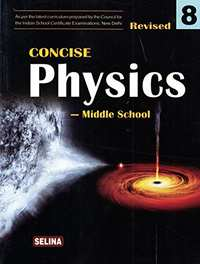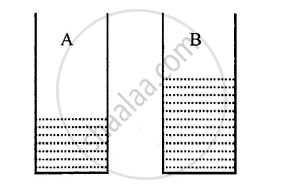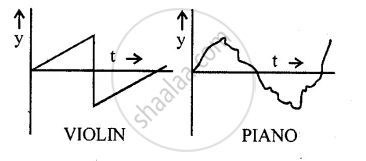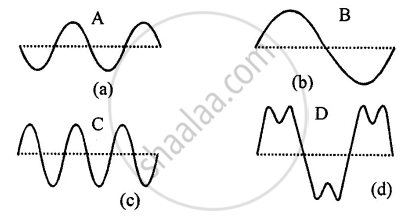Selina solutions for Concise Physics Class 8 ICSE chapter 7 - Sound [Latest edition]

ChaptersChapter 7: Sound

Objective questions [Pages 135 - 136]

Selina solutions for Concise Physics Class 8 ICSE Chapter 7 SoundObjective questions [Pages 135 - 136]

Objective questions | Q 1.01 | Page 135

When sound propagates in air, it does not carry energy with it.

• True

• False

Objective questions | Q 1.02 | Page 135

In a longitudinal wave, compression and rarefaction are formed.

• True

• False

Objective questions | Q 1.03 | Page 135

The distance from one compression to nearest rarefaction is called wavelength.

• True

• False

Objective questions | Q 1.04 | Page 135

The frequency is measured in second.

• True

• False

Objective questions | Q 1.05 | Page 135

The quality of a sound depends on the amplitude of wave.

• True

• False

Objective questions | Q 1.06 | Page 135

The pitch of sound depends on frequency.

• True

• False

Objective questions | Q 1.07 | Page 135

Decibel is the unit of pitch of a sound.

• True

• False

Objective questions | Q 2.01 | Page 135

The time period of a wave is 2 s. Its frequency is ..........

Objective questions | Q 2.02 | Page 135

The pitch of a stringed instrument is increased by .................. tension in string.

Objective questions | Q 2.03 | Page 135

The pitch of a flute is decreased by ................... length of air column.

Objective questions | Q 2.04 | Page 135

Smaller the membrane, .................. is the pitch.

Objective questions | Q 2.05 | Page 135

If a drum is beaten hard, its loudness ................

Objective questions | Q 2.06 | Page 135

A tuning fork produces sound of ................... frequency.

Objective questions | Q 3.01 | Page 135

Match the following

 column A column  B (A) Amplitude (1) frequency (B) Frequency (2) amplitude (C) Loudness (3)maximum displacement on either side (D) Pitch (4) presence of other frequencies (E) Waveform (5) 1/ time period

Select the correct alternative

Objective questions | Q 4.01 | Page 135

Sound can not travel in

• solid

• liquid

• gas

• vacuum

Objective questions | Q 4.02 | Page 135

When sound travels in form of a wave

• the particles of medium move from the source to the listener

• the particles of medium remain stationary

• the particles of medium start vibrating up and down

• the particles of medium transfer energy without leaving their mean positions.

Objective questions | Q 4.03 | Page 135

The safe limit of loudness of audible sound is

• 0 to 80 dB

• above 80 dB

• 120 dB

• above 120 dB

Objective questions | Q 4.04 | Page 136

The unit of loudness is __________

• cm

• second

• hertz

• decibel

Objective questions | Q 4.05 | Page 136

In a piano, pitch is decreased by

• using thicker string

• increasing tension

• reducing length of string

• striking it hard Ans.

Short/Long Answer questions [Pages 136 - 137]

Selina solutions for Concise Physics Class 8 ICSE Chapter 7 SoundShort/Long Answer questions [Pages 136 - 137]

Short/Long Answer questions | Q 1 | Page 136

How does sound travel in air?

Short/Long Answer questions | Q 2 | Page 136

What is longitudinal wave?

Short/Long Answer questions | Q 3 | Page 136

Explain the mechanism of the formation of a longitudinal wave when the source vibrates in the air.

Short/Long Answer questions | Q 4 | Page 136

Define the following terms :
(a) Amplitude
(b) Frequency
(c) Time period.

Short/Long Answer questions | Q 5 | Page 136

Obtain relationship between the time period and frequency.

Short/Long Answer questions | Q 6 | Page 136

Name three characteristics of a musical sound.

Short/Long Answer questions | Q 7 | Page 136

Name the quantity from below which determines the loudness of a sound wave :
(a) Wavelength
(b) Frequency, and
(c) Amplitude

Short/Long Answer questions | Q 8 | Page 136

How is loudness related to the amplitude of wave?

Short/Long Answer questions | Q 9 | Page 136

If the amplitude of a wave is doubled, what will be the effect on its loudness?

Short/Long Answer questions | Q 10 | Page 136

How does the wave pattern of a loud note differ from a soft note? Draw a diagram

Short/Long Answer questions | Q 11 | Page 136

Name the unit in which the loudness of sound is expressed.

Short/Long Answer questions | Q 12 | Page 136

Why is the loudness of sound heard by a plucked wire increased when mounted on a sound board?

Short/Long Answer questions | Q 13 | Page 136

State three factors on which loudness of sound heard by a listener depends.

Short/Long Answer questions | Q 14 | Page 136

What determines the pitch of a sound?

Short/Long Answer questions | Q 15 | Page 136

Name the characteristic of sound related to its frequency

Short/Long Answer questions | Q 16 | Page 136

Name and define the characteristic which enables one to distinguish two sounds of same loudness, but of different frequencies, given by the same instrument.

Short/Long Answer questions | Q 17 | Page 136

Draw a diagram to show the wave pattern of high pitch note and a low pitch note, but of the same loudness.

Short/Long Answer questions | Q 18 | Page 136

How is it possible to detect the filling of a bucket under a water tap by hearing the sound standing at a distance?

Short/Long Answer questions | Q 19 | Page 136

The frequencies of notes given by flute, guitar and trumpet are respectively 400 Hz, 200 Hz and 500 Hz. Which one of these has the highest pitch?

Short/Long Answer questions | Q 20 | Page 136

Figure shows two jars A and B containing water up to different heights. Which will produce sound of higher pitch when air is blown on them?Short/Long Answer questions | Q 21 | Page 136

Two identical guitars are played by two persons to give notes of the same pitch. Will they differ in quantity? Give reason for your answer.

Short/Long Answer questions | Q 22 | Page 136

Two musical notes of the same pitch and same loudness are played on two different instruments. Their wave patterns are as shown in figure.How do they differ in
(a) loudness,
(b) pitch and
(c) quality

Short/Long Answer questions | Q 23 | Page 137

Which characteristics of sound makes it possible to recognize a person by his voice without seeing him?

Short/Long Answer questions | Q 24 | Page 137

State the factors that determine
(a) the pitch of a note.
(b) the loudness of the sound heard.
(c) the quality of the note.

Short/Long Answer questions | Q 25 | Page 137

Name the characteristic of the sound affected due to a change in its

(a) amplitude (b) waveform (c) frequency.

Short/Long Answer questions | Q 26 | Page 137

Figure shows four waves A, B, C, and DName the wave which shows
(a) a note from a musical instrument,
(b) a soft note
(c) a shrill note.

Short/Long Answer questions | Q 27 | Page 137

How is the pitch of sound in a guitar changed if (a) thin wire is used, (b) wire under less tension is used?

Numericals [Page 137]

Selina solutions for Concise Physics Class 8 ICSE Chapter 7 SoundNumericals [Page 137]

Numericals | Q 1 | Page 137

Two waves of the same pitch have amplitudes in the ratio 1: 3 What will be the ratio of their (i) loudness, (ii) pitch?

Numericals | Q 2 | Page 137

Two waves have frequencies 256 Hz and 512 Hz, but same amplitude. Compare their (i) loudness, and (ii) pitch

Chapter 7: SoundSelina solutions for Concise Physics Class 8 ICSE chapter 7 - Sound

Selina solutions for Concise Physics Class 8 ICSE chapter 7 (Sound) include all questions with solution and detail explanation. This will clear students doubts about any question and improve application skills while preparing for board exams. The detailed, step-by-step solutions will help you understand the concepts better and clear your confusions, if any. Shaalaa.com has the CISCE Concise Physics Class 8 ICSE solutions in a manner that help students grasp basic concepts better and faster.

Further, we at Shaalaa.com provide such solutions so that students can prepare for written exams. Selina textbook solutions can be a core help for self-study and acts as a perfect self-help guidance for students.

Concepts covered in Concise Physics Class 8 ICSE chapter 7 Sound are Properties of Sounds, Characteristics of a Sound Wave, Sound, Propagation of Sound, Representation of a Wave, Loudness and Intensity, Pitch (or shrillness) and frequency, Quality (Or Timbre) and Wave Form, Monotone, Production of Sound, Making a Musical Toy.

Using Selina Class 8 solutions Sound exercise by students are an easy way to prepare for the exams, as they involve solutions arranged chapter-wise also page wise. The questions involved in Selina Solutions are important questions that can be asked in the final exam. Maximum students of CISCE Class 8 prefer Selina Textbook Solutions to score more in exam.

Get the free view of chapter 7 Sound Class 8 extra questions for Concise Physics Class 8 ICSE and can use Shaalaa.com to keep it handy for your exam preparation